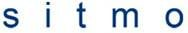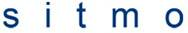# Formulas for sequences

• soothsayer

## Homework Statement

For the following series, write formulas for the sequences an,
Sn and Rn, and find the limits of the sequences as n-->infinityN/A

## The Attempt at a Solution

an is easy, =the limit of which does not exist.

This is where I get stuck, I know Sn=But I don't know how to come up with an actual formula for the sum. Furthermore, I'm not sure I even know what Rn is, a Reimann sum? How do I go about doing that? I can't find anything in my notes.

Maybe it helps if you write it like
$$S_n = \sum_{k = 1}^n \left( - \frac{1}{2} \right)^k$$
(hint: |1/-2| < 1, geometric series).

We cannot smell what Rn is supposed to mean either. Perhaps a recursive formula? Or a Riemann sum? Or a rest term (i.e. defined by $\sum_{k = 0}^\infty a_k = S_n + R_n$) ?

Last edited:
Ooh, thanks for the tip! That does make things a little clearer, I'll try that out. As for what Rn stands for, the problem in the book didn't elaborate, and I don't have the book with me to reference.

If you really want to do this assignment now, I suggest trying the first and last option that I gave.
The recursive formula is quite trivial and will probably take you about 5 second to write down.
The formula for the rest is another nice exercise manipulating geometric series and fractions :-)